table.module-1{width:99.62%;padding:0}table div table+table div table{width:99.62%;float:none;margin-left:auto;margin-right:auto;padding:0}table div table+table div table a{border:0 none;text-decoration:none}table div table+table div table img{width:100%!important;border:0 none;text-decoration:none}table div table+table div table td{width:100%;padding:0}/* styles */

# Thank you for registering for our upcoming Dirty Duathlon or Trail Run at Rope Mill Park on November 13th. The following outlines the procedures that will be implemented for the 2021 edition of this event. Knowing these procedures will help us ensure we have a safe and smooth event for everyone.

## CHECK-IN

▪ Waivers should be printed at home, filled out ahead of time, and brought to packet pickup CLICK HERE FOR EVENT WAIVER You may pick up someone else's number ONLY IF YOU BRING A WAIVER SIGNED BY THEM.
* Optional early packet pickup is at OUTSPOKIN BICYCLES: 3422 Sixes Rd #110, Canton, GA 30114 on Thursday 11/11 from 4 to 7 pm. You will receive your race bib and swag at this time. If you check in early, you can go straight to the start on Saturday morning. (RECOMMENDED)
▪ No race day registrations or participant swaps. If you want to change teammates or need to switch from team to solo, you need to contact me via email no later than 11/8 and we'll get you taken care of. No changes after 11/8.
▪ Saturday Check-in hours are from 7 am to 8:30 am on Saturday morning. When you arrive, parking volunteers will direct you where to park, which will be in one of 3 locations depending on when you arrive. If you arrive later, be prepared to park at the Kirk Rudy office and jog/ride the 1/2 mile down the road to the start. Leave yourself enough time to check-in and get to the start. We will have a bag drop area near the pavilion for trail run only participants or you can leave items in the transition area so you do not have to go back to your car until after the race. PRO TIP: PUT ALL YOUR GEAR IN A BACKPACK SO ITS EASY TO CARRY
▪ Proceed to appropriate check-in line with waiver. You will receive your race bib and swag at this time.
▪ Port-o-lets as well as permanent bathrooms with running water will be available at this venue.
 ▪ Waivers should be printed at home, filled out ahead of time, and brought to packet pickup CLICK HERE FOR EVENT WAIVER You may pick up someone else's number ONLY IF YOU BRING A WAIVER SIGNED BY THEM. * Optional early packet pickup is at OUTSPOKIN BICYCLES: 3422 Sixes Rd #110, Canton, GA 30114 on Thursday 11/11 from 4 to 7 pm. You will receive your race bib and swag at this time. If you check in early, you can go straight to the start on Saturday morning. (RECOMMENDED)
 ▪ No race day registrations or participant swaps. If you want to change teammates or need to switch from team to solo, you need to contact me via email no later than 11/8 and we'll get you taken care of. No changes after 11/8.
 ▪ Saturday Check-in hours are from 7 am to 8:30 am on Saturday morning. When you arrive, parking volunteers will direct you where to park, which will be in one of 3 locations depending on when you arrive. If you arrive later, be prepared to park at the Kirk Rudy office and jog/ride the 1/2 mile down the road to the start. Leave yourself enough time to check-in and get to the start. We will have a bag drop area near the pavilion for trail run only participants or you can leave items in the transition area so you do not have to go back to your car until after the race. PRO TIP: PUT ALL YOUR GEAR IN A BACKPACK SO ITS EASY TO CARRY
 ▪ Proceed to appropriate check-in line with waiver. You will receive your race bib and swag at this time.
 ▪ Port-o-lets as well as permanent bathrooms with running water will be available at this venue.

## RACE PROCEDURES

▪ We will have a brief pre-race meeting at 8:35 am before staging starts.
▪ RACERS ONLY in the transition area. It wil be crowded in the Transition Area, especially pre-race as racers will be setting up their gear. Please have your family members hang out elsewhere to reduce crowding.
▪ Make sure the tyvek race bib is pinned on your FRONT. Those in the trail run only will have a tyvek bib with timing chip. Those in the Duathlon will NOT have a timing chip on their running bib. All duathlon timing is done with the cardboard bike number plate, which gets mounted on your handlebars. If you are a team in the duathlon, the rider gets the cardboard bike plate and the runner wears the pin-on tyvek race number. Solo duathlon participants wear the pin-on tyvek race number as well as mount the cardboard number plate to their bike handlebars.
▪ If you are on a 3 person team, you will need to transfer the bike plate to the second rider's bike while your runner is completing the run segment.
▪ We will have multiple start waves for the trail run and duathlon beginning at 8:55 am. YOU CAN VIEW YOUR START WAVE HERE. Your wave number will be written on your number plate.
▪ There is an Aid Station with water, Hammer HEED and Hammer Gels on the run course at approximately mile 1 and mile 2. There will also be Hammer Gel and Fizz included in each race bag that you can take with you.
▪ Do not litter anywhere on the course - penalty is a DQ
▪ Course will be well marked with pink ribbons and pink/black arrow signs at turns. Pink ribbons indicate the direction you want to head and are also used to designate that you are on-course if it has been a while in between turns. Course marshals will be positioned throughout the course for your safety.
▪ Duathlon course is bike the Mill Trails CW, run Explorer Trails CCW, then bike Avalanche Trails CCW. Trail run only will run the Explorer Trails CCW.
▪ Announce if you need to pass runners/riders ahead by saying NEED TO PASS, RIDER BACK or ON YOU LEFT/RIGHT and give them a chance to move over a bit BEFORE YOU PASS THEM. It is the passing riders responsibility to execute a clean pass. BE NICE OUT THERE and work together.
▪ COURSE MAPS AND DESCRIPTIONS CAN BE FOUND HERE
▪ Snacks and drinks will be available for all participants at the pavilion post-race.
 ▪ We will have a brief pre-race meeting at 8:35 am before staging starts.
 ▪ RACERS ONLY in the transition area. It wil be crowded in the Transition Area, especially pre-race as racers will be setting up their gear. Please have your family members hang out elsewhere to reduce crowding.
 ▪ Make sure the tyvek race bib is pinned on your FRONT. Those in the trail run only will have a tyvek bib with timing chip. Those in the Duathlon will NOT have a timing chip on their running bib. All duathlon timing is done with the cardboard bike number plate, which gets mounted on your handlebars. If you are a team in the duathlon, the rider gets the cardboard bike plate and the runner wears the pin-on tyvek race number. Solo duathlon participants wear the pin-on tyvek race number as well as mount the cardboard number plate to their bike handlebars.
 ▪ If you are on a 3 person team, you will need to transfer the bike plate to the second rider's bike while your runner is completing the run segment.
 ▪ We will have multiple start waves for the trail run and duathlon beginning at 8:55 am. YOU CAN VIEW YOUR START WAVE HERE. Your wave number will be written on your number plate.
 ▪ There is an Aid Station with water, Hammer HEED and Hammer Gels on the run course at approximately mile 1 and mile 2. There will also be Hammer Gel and Fizz included in each race bag that you can take with you.
 ▪ Do not litter anywhere on the course - penalty is a DQ
 ▪ Course will be well marked with pink ribbons and pink/black arrow signs at turns. Pink ribbons indicate the direction you want to head and are also used to designate that you are on-course if it has been a while in between turns. Course marshals will be positioned throughout the course for your safety.
 ▪ Duathlon course is bike the Mill Trails CW, run Explorer Trails CCW, then bike Avalanche Trails CCW. Trail run only will run the Explorer Trails CCW.
 ▪ Announce if you need to pass runners/riders ahead by saying NEED TO PASS, RIDER BACK or ON YOU LEFT/RIGHT and give them a chance to move over a bit BEFORE YOU PASS THEM. It is the passing riders responsibility to execute a clean pass. BE NICE OUT THERE and work together.
 ▪ Snacks and drinks will be available for all participants at the pavilion post-race.

## COURSE FLOW/TRANSITION AREA SETUP

Solos: ensure your pin-on number is pinned to the front of your body and the cardboard number is mounted on the handlebar of your bike. Teams: ensure pin-on number is pinned on front of RUNNER, and cardboard number is mounted on handlebar of bike for RIDER. 3 Person Teams: ensure you switch the bike number to the second rider's bike while your runner is out.

Duathlon participants will start the race on bike. There will be start corrals marked along the concrete path that correspond to your start wave. You need to exit the Transition Area by 8:45 and head to start staging. YOU CAN VIEW YOUR START WAVE HERE. Your wave number will be written on your number plate. You will be released in waves and complete the Mill Trails CW.

As riders return from Bike Leg 1 on the Mill Trails, you will need to dismount your bike in the grass just past the bridge and run through the small ditch into the Transition Area. There will be signs that say DISMOUNT HERE. You must dismount and run your bike into the TA.

Once in the transition area, solos will rack their bike and then head out the back of the TA onto Explorer and complete the run loop CCW. For teams, the rider needs to physically tag their runner before the runner can head out. Teams should also re-rack their bikes while waiting. You must keep the aisles of the TA clear - do not block aisles with bikes.

Once back from the run, solos retrieve their bike from the racks and they need to RUN it out of the TA (no riding in TA) across the small wooden bridge, back towards the finish arch. Once across the bridge, you can get back on the bike and ride through the finish arch, through the circle parking lot and onto Avalanche Trail CCW (This will all be marked). For teams, the returning runner needs to tag the rider before the rider can leave the TA, also RUNNING their bike across the wooden bridge. The main aisle of the TA leading to the wooden bridge needs to be kept clear so Team riders should not unrack their bike until they see their runner heading back in.

Once riders complere bike leg 2 on Avalanche CCW, they will finish via the big hill behind the pavilion and ride through the finish.

No spectators in the TA - please encourage them to watch elsewhere.

Once solos/teams finish the race, you may not exit TA over the wooden bridge when done as you will interfere with finishing traffic. You must exit out the back of TA or through the woods on side.

## RESULTS/AWARDS

▪ Finish times wlll be uploaded to our resutls website, mgaresults.com , so you can view them on your mobile device from anywhere at the venue.
▪ Trail Run awards are at 10:30 am Duathlon awards will be at approximately 11:30 am, assuming we have enough finishers in. AWARDS WILL NOT BE MAILED - THEY MUST BE PICKED UP AT THE RACE
 ▪ Finish times wlll be uploaded to our resutls website, mgaresults.com , so you can view them on your mobile device from anywhere at the venue.
 ▪ Trail Run awards are at 10:30 am Duathlon awards will be at approximately 11:30 am, assuming we have enough finishers in. AWARDS WILL NOT BE MAILED - THEY MUST BE PICKED UP AT THE RACE
 table div table+table+table+table div table{width:100%;padding:0}table div table+table+table+table div table img{width:96.23%;padding:0;float:none}table div table+table+table+table div table td{width:100%;padding:0 1.88% 18px}/* styles */## Martha's Coffee On Site!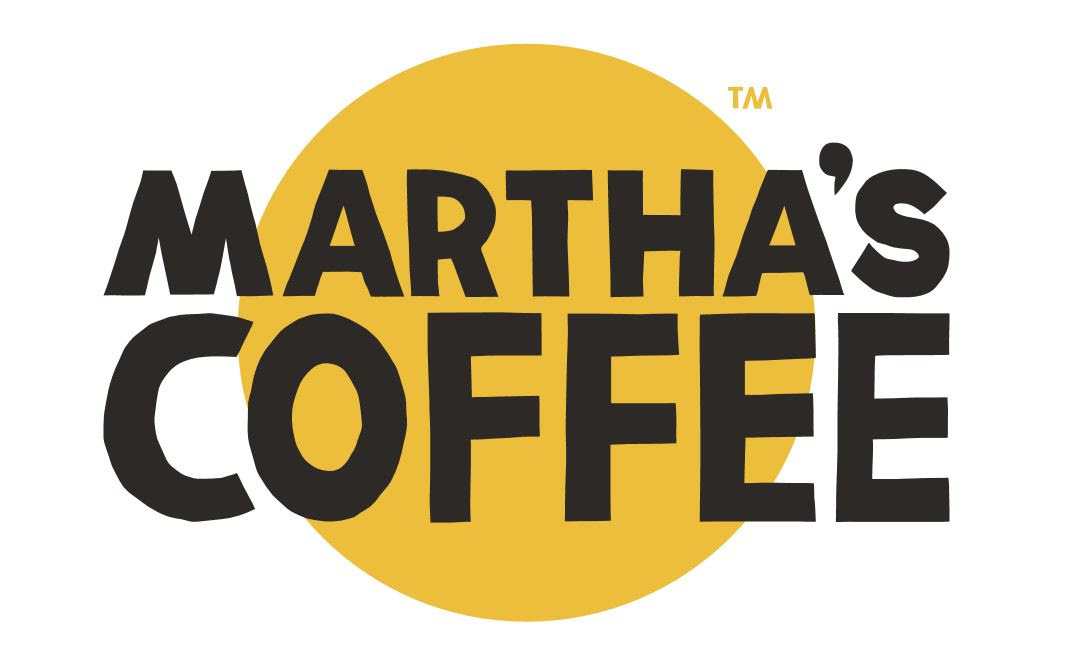Its usually chilly down by the river first thing in the morning, but Martha's Coffee will be on site for coffee, hot chocolate and tea purchases. They can take cash or cards.

 table div table+table+table+table+table+table+table div table{width:100%;padding:0}table div table+table+table+table+table+table+table div table img{width:96.23%;padding:0;float:none}table div table+table+table+table+table+table+table div table td{width:100%;padding:0 1.88% 18px}/* styles */## Hoodies, Goat Gear and Clearance Shirts Available at this event - CASH or VENMO ONLY

 /* styles */ We have an entire year's worth of clearance race shirts that we want to find homes for. Help me downsize and pick up a shirt or four. \$3 each or 2 for \$5. We have both men's and ladies sizes available in various race designs. We have a few hoodies left in assorted sizes/colors for \$20 each. We will also have our hats, visors and thermal beanies for sale. All are \$15 each. Thermal headbands are \$12 each. Cash or Venmo only on merchandise sales at this event.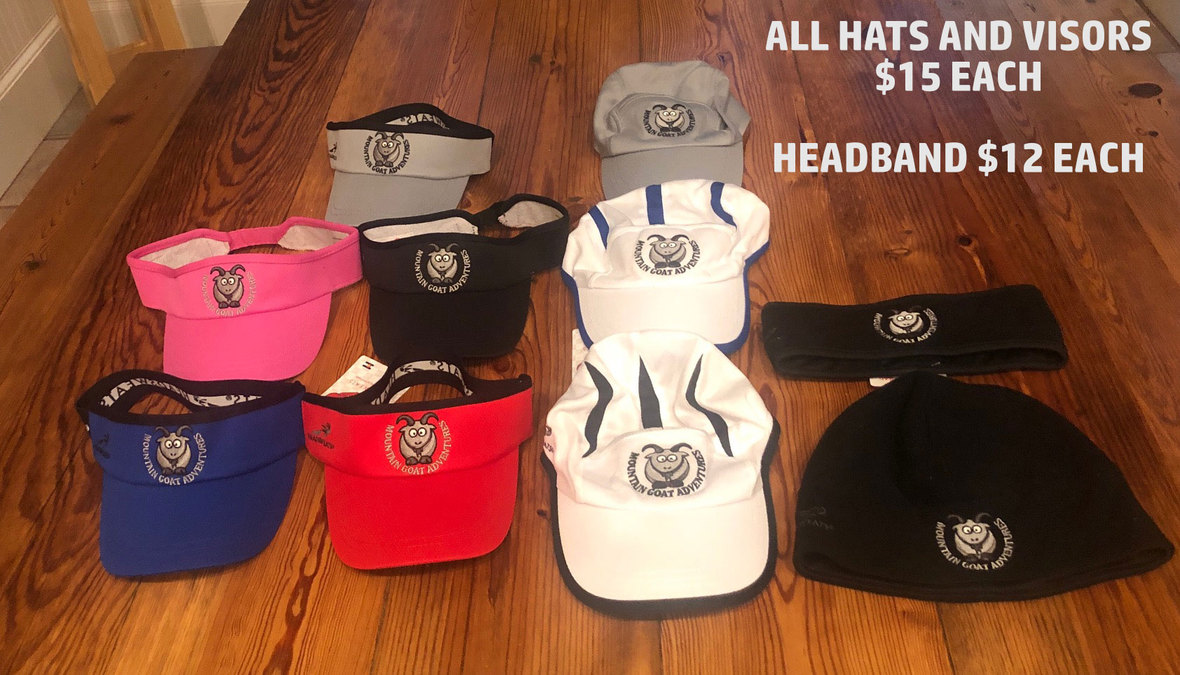table div table+table+table+table+table+table+table+table+table+table+table div table{width:100%;padding:0}table div table+table+table+table+table+table+table+table+table+table+table div table img{width:96.23%;padding:0;float:none}table div table+table+table+table+table+table+table+table+table+table+table div table td{width:100%;padding:0 1.88% 18px}/* styles */# Hammer Nutrition endurance fuels will be on the course at the 2021 Rope Mill Dirty Duathlon!

Hammer has been supplying athletes with natural products free of added sugars, artificial colors, flavors, chemicals, and preservatives for over 30 years.

## ROPE MILL DUATHLON TIP #4: BEFORE WORKOUTS AND RACES, CONSUME 300-400 CALORIES. COMPLETE YOUR MEAL 3 HOURS BEFORE YOU START EXERCISE

To perform and feel your best during races or workouts, consume no more than 300-400 calories. Choose easily digested, complex carbohydrates, along with a small amount of protein and a little healthy fat. Avoid fiber, simple sugar, and acidic foods. Finish your meal 3 hours before exercise. Eating within 3 hours can seriously hurt your performance by 1) reducing the conversion of fats to fuels, and 2) accelerating glycogen depletion.

Tip: If your race is early, don't sacrifice sleep to eat! Instead, consume a small amount of supplemental fuel, such as one Hammer Gel, about 5 minutes before starting.

## ROPE MILL DUATHLON TIP #5: REPLENISH YOUR BODY WITH CARBOHYDRATES AND PROTEIN AS SOON AS POSSIBLE AFTER EACH EXERCISE SESSION, IDEALLY WITHIN THE FIRST 30-60 MINUTES.

What you do immediately after your workout is just as important as your workout itself! "Refill the tank" as soon as possible (ideally within the first 60 minutes) to fully replenish glycogen and build and repair muscle tissue. Consume 30-60 grams of complex carbohydrates and 10-20 grams of protein. Recoverite supplies both in the ideal 3:1 ratio. Also, be sure to take antioxidants after exercise to neutralize cell-damaging free radicals and speed recovery.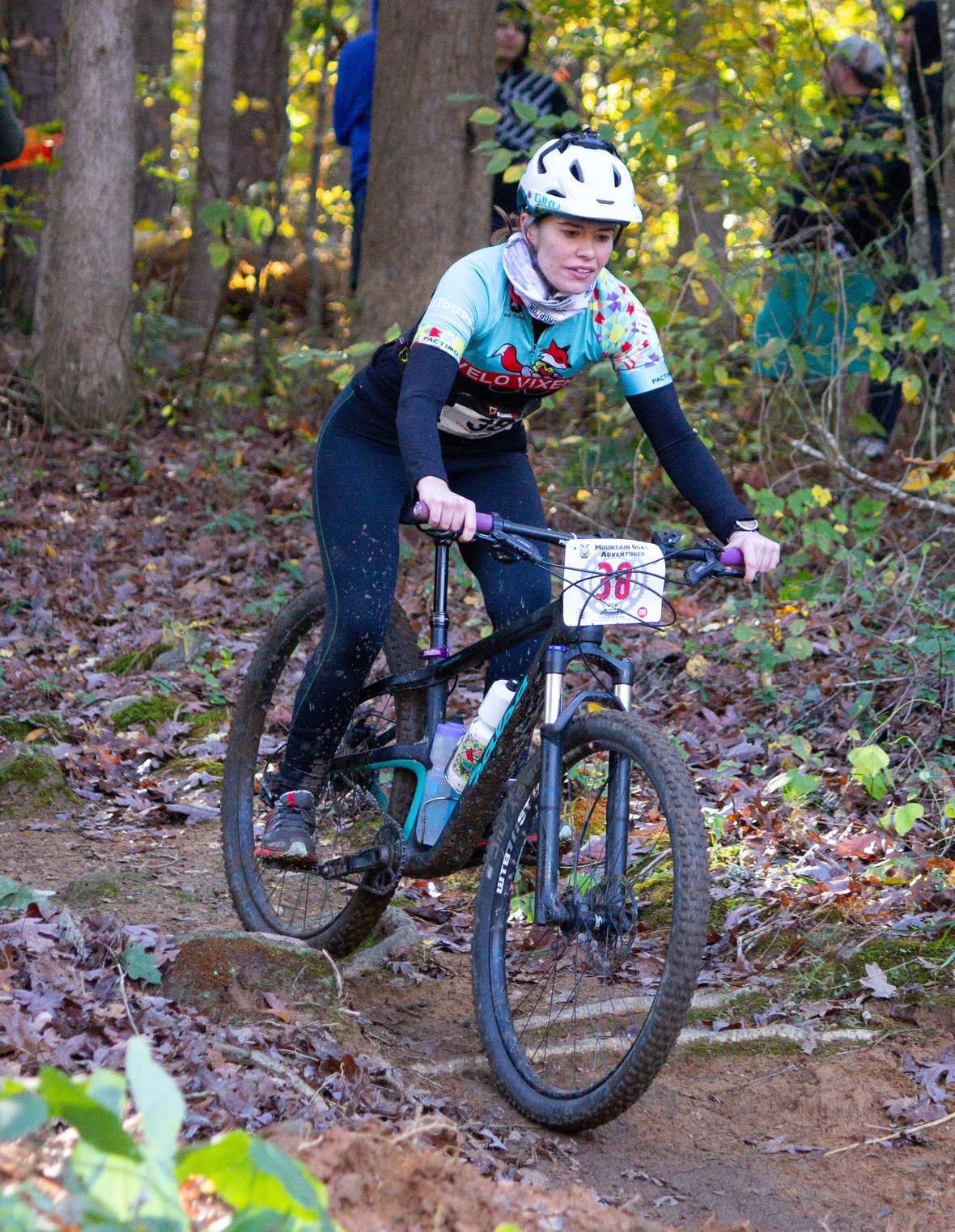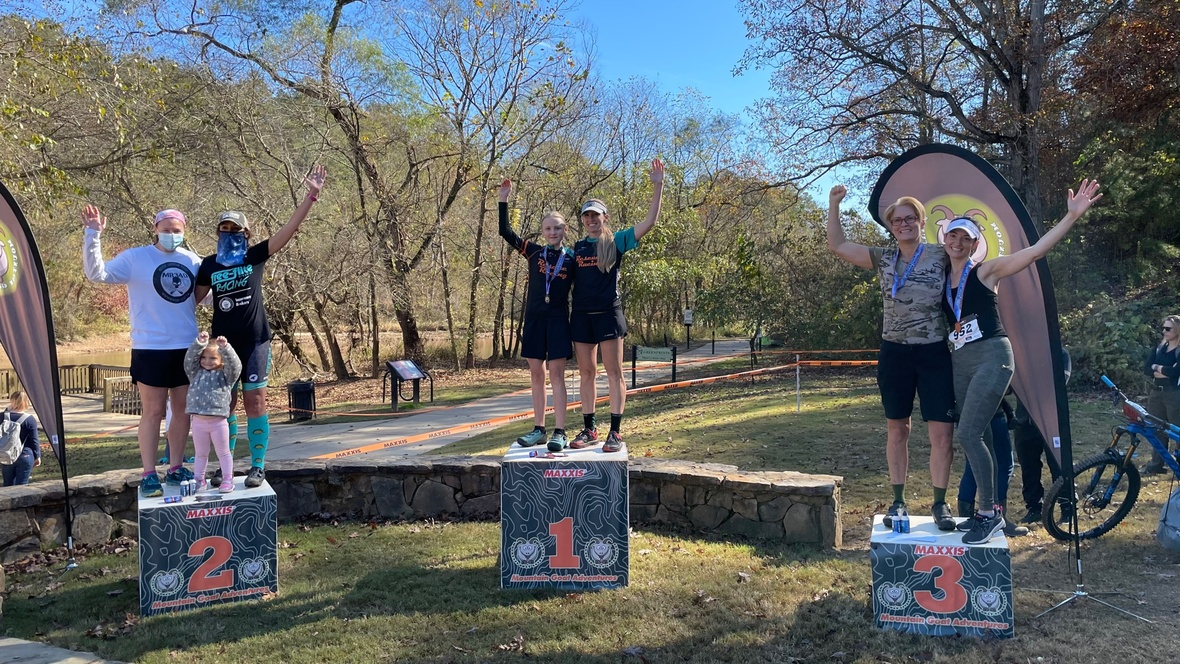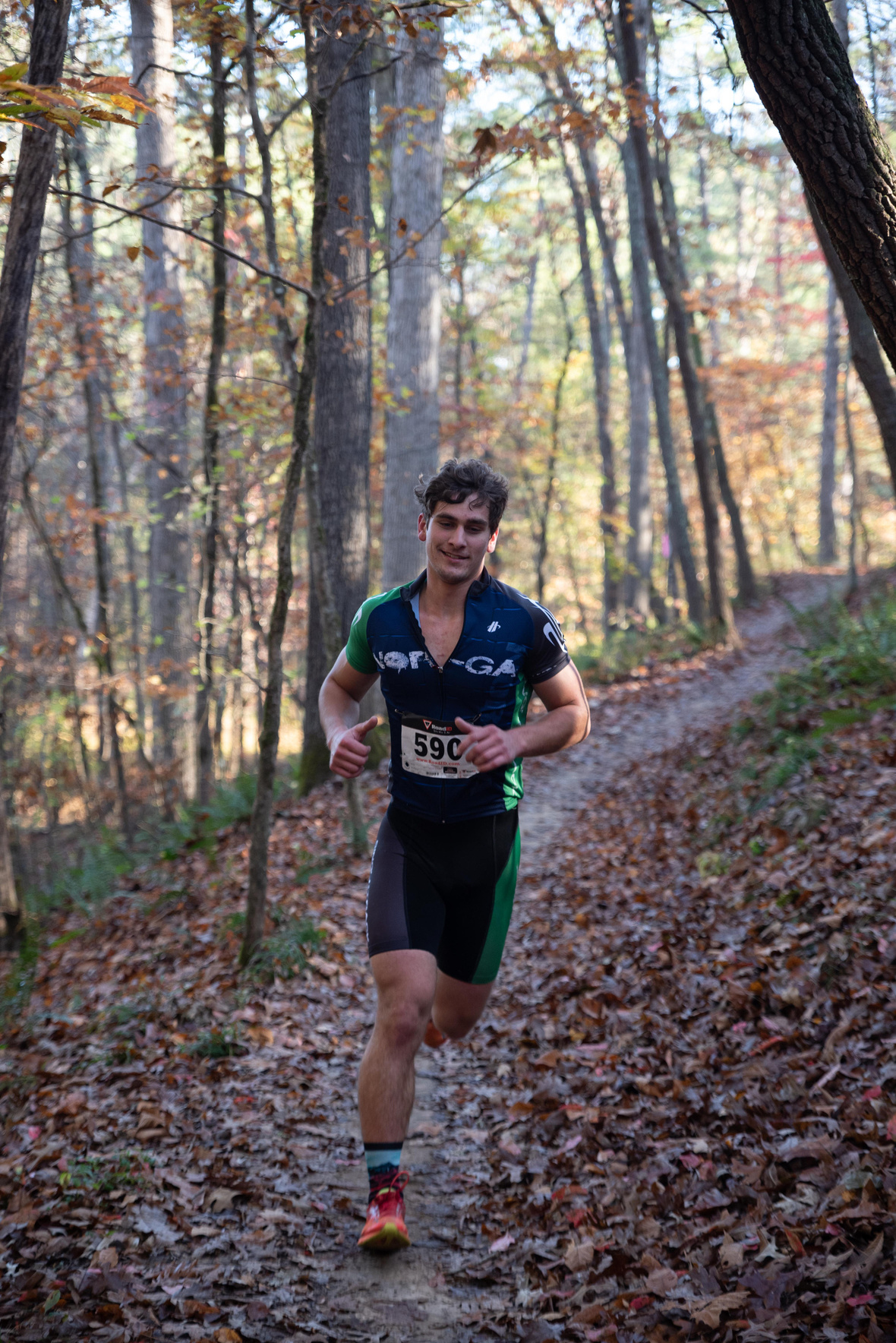table div table+table+table+table+table+table+table+table+table+table+table+table+table+table+table+table div table{width:100%;padding:0}table div table+table+table+table+table+table+table+table+table+table+table+table+table+table+table+table div table img{width:96.23%;padding:0;float:none}table div table+table+table+table+table+table+table+table+table+table+table+table+table+table+table+table div table td{width:100%;padding:0 1.88% 18px}/* styles */## Special Thanks to our Sponsors!

 table.module-17{width:99.62%;padding:0}table div table+table+table+table+table+table+table+table+table+table+table+table+table+table+table+table+table+table div table{width:99.62%;float:none;margin-left:auto;margin-right:auto;padding:0}table div table+table+table+table+table+table+table+table+table+table+table+table+table+table+table+table+table+table div table a{border:0 none;text-decoration:none}table div table+table+table+table+table+table+table+table+table+table+table+table+table+table+table+table+table+table div table img{width:100%!important;border:0 none;text-decoration:none}table div table+table+table+table+table+table+table+table+table+table+table+table+table+table+table+table+table+table div table td{width:100%;padding:0}/* styles */
 table.module-18{width:75.47%;padding:0}table div table+table+table+table+table+table+table+table+table+table+table+table+table+table+table+table+table+table+table div table{width:75.47%;float:none;margin-left:auto;margin-right:auto;padding:0}table div table+table+table+table+table+table+table+table+table+table+table+table+table+table+table+table+table+table+table div table a{border:0 none;text-decoration:none}table div table+table+table+table+table+table+table+table+table+table+table+table+table+table+table+table+table+table+table div table img{width:100%!important;border:0 none;text-decoration:none}table div table+table+table+table+table+table+table+table+table+table+table+table+table+table+table+table+table+table+table div table td{width:100%;padding:0}/* styles */
 table div table+table+table+table+table+table+table+table+table+table+table+table+table+table+table+table+table+table+table+table div table{width:100%;padding:0}table div table+table+table+table+table+table+table+table+table+table+table+table+table+table+table+table+table+table+table+table div table table{padding:0;float:left!important;width:36.603%!important}table div table+table+table+table+table+table+table+table+table+table+table+table+table+table+table+table+table+table+table+table div table table+table td,table div table+table+table+table+table+table+table+table+table+table+table+table+table+table+table+table+table+table+table+table div table td{padding-left:0;padding-right:0}table div table+table+table+table+table+table+table+table+table+table+table+table+table+table+table+table+table+table+table+table div table table td{padding-left:0;padding-right:20px}table div table+table+table+table+table+table+table+table+table+table+table+table+table+table+table+table+table+table+table+table div table table+table{float:left!important;width:63.397%!important}/* styles */
 table div table+table+table+table+table+table+table+table+table+table+table+table+table+table+table+table+table+table+table+table+table div table{width:100%;padding:0}table div table+table+table+table+table+table+table+table+table+table+table+table+table+table+table+table+table+table+table+table+table div table table{padding:0;float:left!important;width:37.547%!important}table div table+table+table+table+table+table+table+table+table+table+table+table+table+table+table+table+table+table+table+table+table div table table+table td,table div table+table+table+table+table+table+table+table+table+table+table+table+table+table+table+table+table+table+table+table+table div table td{padding-left:0;padding-right:0}table div table+table+table+table+table+table+table+table+table+table+table+table+table+table+table+table+table+table+table+table+table div table table td{padding-left:0;padding-right:20px}table div table+table+table+table+table+table+table+table+table+table+table+table+table+table+table+table+table+table+table+table+table div table table+table{float:left!important;width:62.453%!important}/* styles */
 table div table+table+table+table+table+table+table+table+table+table+table+table+table+table+table+table+table+table+table+table+table+table div table{width:100%;padding:0}table div table+table+table+table+table+table+table+table+table+table+table+table+table+table+table+table+table+table+table+table+table+table div table table{padding:0;float:left!important;width:25.66%!important}table div table+table+table+table+table+table+table+table+table+table+table+table+table+table+table+table+table+table+table+table+table+table div table table+table+table td,table div table+table+table+table+table+table+table+table+table+table+table+table+table+table+table+table+table+table+table+table+table+table div table td{padding-left:0;padding-right:0}table div table+table+table+table+table+table+table+table+table+table+table+table+table+table+table+table+table+table+table+table+table+table div table table td,table div table+table+table+table+table+table+table+table+table+table+table+table+table+table+table+table+table+table+table+table+table+table div table table+table td{padding-left:0;padding-right:20px}table div table+table+table+table+table+table+table+table+table+table+table+table+table+table+table+table+table+table+table+table+table+table div table table+table{float:left!important;width:54.528%!important}table div table+table+table+table+table+table+table+table+table+table+table+table+table+table+table+table+table+table+table+table+table+table div table table+table+table{float:left!important;width:19.811999999999998%!important}/* styles */
 table div table+table+table+table+table+table+table+table+table+table+table+table+table+table+table+table+table+table+table+table+table+table+table div table{width:100%;padding:0}table div table+table+table+table+table+table+table+table+table+table+table+table+table+table+table+table+table+table+table+table+table+table+table div table table{padding:0;float:left!important;width:56.415%!important}table div table+table+table+table+table+table+table+table+table+table+table+table+table+table+table+table+table+table+table+table+table+table+table div table table+table td,table div table+table+table+table+table+table+table+table+table+table+table+table+table+table+table+table+table+table+table+table+table+table+table div table td{padding-left:0;padding-right:0}table div table+table+table+table+table+table+table+table+table+table+table+table+table+table+table+table+table+table+table+table+table+table+table div table table td{padding-left:0;padding-right:20px}table div table+table+table+table+table+table+table+table+table+table+table+table+table+table+table+table+table+table+table+table+table+table+table div table table+table{float:left!important;width:43.585%!important}/* styles */
 table div table+table+table+table+table+table+table+table+table+table+table+table+table+table+table+table+table+table+table+table+table+table+table+table div table{width:100%;padding:0}table div table+table+table+table+table+table+table+table+table+table+table+table+table+table+table+table+table+table+table+table+table+table+table+table div table table{padding:0;float:left!important;width:36.037%!important}table div table+table+table+table+table+table+table+table+table+table+table+table+table+table+table+table+table+table+table+table+table+table+table+table div table table+table td,table div table+table+table+table+table+table+table+table+table+table+table+table+table+table+table+table+table+table+table+table+table+table+table+table div table td{padding-left:0;padding-right:0}table div table+table+table+table+table+table+table+table+table+table+table+table+table+table+table+table+table+table+table+table+table+table+table+table div table table td{padding-left:0;padding-right:20px}table div table+table+table+table+table+table+table+table+table+table+table+table+table+table+table+table+table+table+table+table+table+table+table+table div table table+table{float:left!important;width:63.963%!important}/* styles */
 table div table+table+table+table+table+table+table+table+table+table+table+table+table+table+table+table+table+table+table+table+table+table+table+table+table div table{width:100%;padding:0}table div table+table+table+table+table+table+table+table+table+table+table+table+table+table+table+table+table+table+table+table+table+table+table+table+table div table table{padding:0;float:left!important;width:34.528%!important}table div table+table+table+table+table+table+table+table+table+table+table+table+table+table+table+table+table+table+table+table+table+table+table+table+table div table table+table+table td,table div table+table+table+table+table+table+table+table+table+table+table+table+table+table+table+table+table+table+table+table+table+table+table+table+table div table td{padding-left:0;padding-right:0}table div table+table+table+table+table+table+table+table+table+table+table+table+table+table+table+table+table+table+table+table+table+table+table+table+table div table table td,table div table+table+table+table+table+table+table+table+table+table+table+table+table+table+table+table+table+table+table+table+table+table+table+table+table div table table+table td{padding-left:0;padding-right:20px}table div table+table+table+table+table+table+table+table+table+table+table+table+table+table+table+table+table+table+table+table+table+table+table+table+table div table table+table{float:left!important;width:42.075%!important}table div table+table+table+table+table+table+table+table+table+table+table+table+table+table+table+table+table+table+table+table+table+table+table+table+table div table table+table+table{float:left!important;width:23.39699999999999%!important}/* styles */
 table div table+table+table+table+table+table+table+table+table+table+table+table+table+table+table+table+table+table+table+table+table+table+table+table+table+table div table{width:100%;padding:0}table div table+table+table+table+table+table+table+table+table+table+table+table+table+table+table+table+table+table+table+table+table+table+table+table+table+table div table table{padding:0;float:left!important;width:35.66%!important}table div table+table+table+table+table+table+table+table+table+table+table+table+table+table+table+table+table+table+table+table+table+table+table+table+table+table div table table+table td,table div table+table+table+table+table+table+table+table+table+table+table+table+table+table+table+table+table+table+table+table+table+table+table+table+table+table div table td{padding-left:0;padding-right:0}table div table+table+table+table+table+table+table+table+table+table+table+table+table+table+table+table+table+table+table+table+table+table+table+table+table+table div table table td{padding-left:0;padding-right:20px}table div table+table+table+table+table+table+table+table+table+table+table+table+table+table+table+table+table+table+table+table+table+table+table+table+table+table div table table+table{float:left!important;width:64.34%!important}/* styles */
 table.module-26{width:99.25%;padding:0}table div table+table+table+table+table+table+table+table+table+table+table+table+table+table+table+table+table+table+table+table+table+table+table+table+table+table+table div table{width:99.25%;float:none;margin-left:auto;margin-right:auto;padding:0}table div table+table+table+table+table+table+table+table+table+table+table+table+table+table+table+table+table+table+table+table+table+table+table+table+table+table+table div table a{border:0 none;text-decoration:none}table div table+table+table+table+table+table+table+table+table+table+table+table+table+table+table+table+table+table+table+table+table+table+table+table+table+table+table div table img{width:100%!important;border:0 none;text-decoration:none}table div table+table+table+table+table+table+table+table+table+table+table+table+table+table+table+table+table+table+table+table+table+table+table+table+table+table+table div table td{width:100%;padding:0}/* styles */